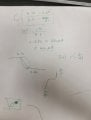# How to calculate the discharged current of a capacitor from the discharge curve?

Joined Jun 15, 2021
13
I observed the discharge of a capacitor on an energy harvester chip via an oscilloscope. The Output was used to power a BLE module.I know the capacitor value, discharge time, and the top and bottom voltage. I don't think I know my load resistor because it is a bit complicated to measure.

Is there a way to measure the total discharged current?

I know the capacitance C (1000uF) then the slope of the curve is proportional to the discharge current.

i(t) = C dvdt

However, I want to know the total current discharged by the capacitor or the entire power (Calculation)? How to do it?

#### BobTPH

Joined Jun 5, 2013
3,773
The discharge looks pretty close to linear. You can compute the current directly from the equation:

I = C dv/dt

Get dv and dt from your scope trace and do the math.

Bob

Joined Jun 15, 2021
13
The discharge looks pretty close to linear. You can compute the current directly from the equation:

I = C dv/dt

Get dv and dt from your scope trace and do the math.

Bob
dv/dt can be calculated by taking any two points on the curve and calculating the slope. right? However, would that accommodate for the entire power used across the slope? let's say I get a number for current, what do I multiply it by to get the power used by the full discharge?

#### Ian0

Joined Aug 7, 2020
3,463
Q=CV

So that charge at the beginning Q1 = C *V1 (the Voltage at the beginning)
Q2 = C*V2 (the voltage at the end)

So the average current is the change in charge divided by the change in time.
I = ΔQ/Δt

And if you want to know the energy used.
E = ½CV^2
so you can work out the energy at the beginning and the energy at the end, and therefore the energy used.
And the power is the rate of usage of energy

Joined Jun 15, 2021
13
Q=CV

So that charge at the beginning Q1 = C *V1 (the Voltage at the beginning)
Q2 = C*V2 (the voltage at the end)

So the average current is the change in charge divided by the change in time.
I = ΔQ/Δt

And if you want to know the energy used.
E = ½CV^2
so you can work out the energy at the beginning and the energy at the end, and therefore the energy used.
And the power is the rate of usage of energyI made the calculation and it is up to 280 uA. However, I remember when I power my chip directly with no capacitor. the current consumption fluctuates between two values due to the duty cycle of the transmitted information. So, does it make sense that my slope is linear and I have one value current representation for current consumption?

#### Ian0

Joined Aug 7, 2020
3,463
280uA is the average figure.
I can't see the timebase in the picture, so I don't know how long the discharge takes.
If you magnify it you should see periods of steeper slope where the current consumption is higher.